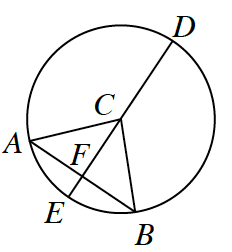### Home > GC > Chapter 8 > Lesson 8.3.3 > Problem8-108

8-108.Larry started to set up a proof to show that if $\overline{AB} \bot \overline{DE}$ and $\overline{DE}$ is a diameter of $\odot C$, then $\overline{AF} \cong \overline{FB}$. Examine his work below. Then complete his missing statements and reasons.

 Statements Reasons $\overline{AB} \bot \overline{DE}$  and is a diameter of $\odot C$. $\angle AFC$ and $\angle BFC$ are right angles. $FC = FC$ $AC = BC$ Definition of a Circle (radii must be equal) $\text{HL}\cong$ $\overline{AF}\cong\overline{FB}$

Click the check boxes as they appear in order to complete the proof.

1. Given

2. Definition of perpendicular

3. Reflexive property

5. $\Delta AFC \cong \Delta BFC$

6. $\cong \Delta \rightarrow \: \cong \text { parts}$